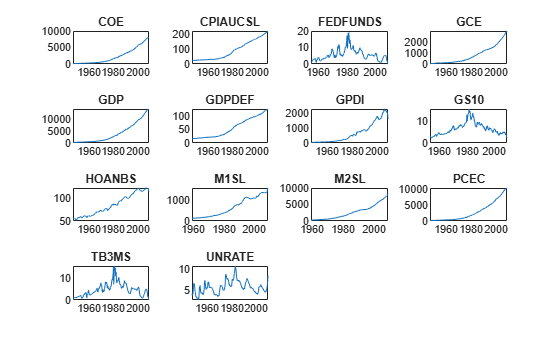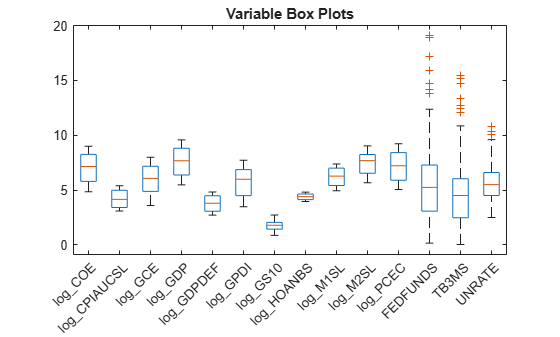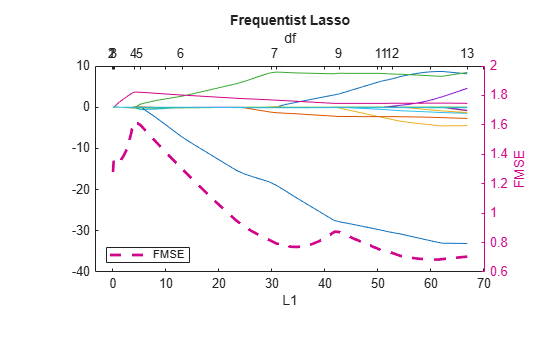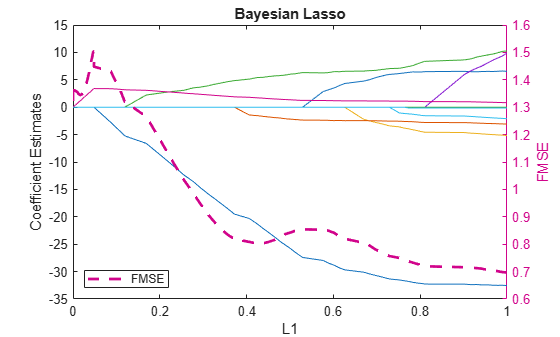# Bayesian Lasso Regression

This example shows how to perform variable selection by using Bayesian lasso regression.

Lasso regression is a linear regression technique that combines regularization and variable selection. Regularization helps prevent overfitting by decreasing the magnitude of the regression coefficients. The frequentist view of lasso regression differs from that of other regularization techniques, such as, ridge regression, because lasso attributes a value of exactly 0 to regression coefficients corresponding to predictors that are insignificant or redundant.

Consider this multiple linear regression model:•is a vector of n responses.

•is a matrix of p corresponding observed predictor variables.

•is a vector of p regression coefficients.

•is the intercept.

•is a length n column vector of ones.

•is a random vector of iid Gaussian disturbances with a mean of 0 and variance of.

The objective function of the frequentist view of lasso regression isis the penalty (or shrinkage) term and, unlike other parameters, is not fit to the data. You must specify a value forbefore estimation, and a best practice is to tune it. After you specify a value,is minimized with respect to the regression coefficients. The resulting coefficients are the lasso estimates. For more details on the frequentist view of lasso regression, see .

For this example, consider creating a predictive linear model for the US unemployment rate. You want a model that generalizes well. In other words, you want to minimize the model complexity by removing all redundant predictors and all predictors that are uncorrelated with the unemployment rate.

Load the US macroeconomic data set Data_USEconModel.mat.

load Data_USEconModel 

The data set includes the MATLAB® timetable DataTable, which contains 14 variables measured from Q1 1947 through Q1 2009; UNRATE is the US unemployment rate. For more details, enter Description at the command line.

Plot all series in the same figure, but in separate subplots.

figure; for j = 1:size(DataTable,2) subplot(4,4,j) plot(DataTable.Time,DataTable{:,j}); title(DataTable.Properties.VariableNames(j)); endAll series except FEDFUNDS, GS10, TB3MS, and UNRATE appear to have an exponential trend.

Apply the log transform to those variables that have an exponential trend.

hasexpotrend = ~ismember(DataTable.Properties.VariableNames,... ["FEDFUNDS" "GD10" "TB3MS" "UNRATE"]); DataTableLog = varfun(@log,DataTable,'InputVariables',... DataTable.Properties.VariableNames(hasexpotrend)); DataTableLog = [DataTableLog DataTable(:,DataTable.Properties.VariableNames(~hasexpotrend))]; 

DataTableLog is a timetable like DataTable, but those variables with an exponential trend are on the log scale.

Coefficients that have relatively large magnitudes tend to dominate the penalty in the lasso regression objective function. Therefore, it is important that variables have a similar scale when you implement lasso regression. Compare the scales of the variables in DataTableLog by plotting their box plots on the same axis.

figure; boxplot(DataTableLog.Variables,'Labels',DataTableLog.Properties.VariableNames); h = gca; h.XTickLabelRotation = 45; title('Variable Box Plots');The variables have fairly similar scales.

To determine how well time series models generalize, follow this procedure:

1. Partition the data into estimation and forecast samples.

2. Fit the models to the estimation sample.

3. Use the fitted models to forecast responses into the forecast horizon.

4. Estimate the forecast mean squared error (FMSE) for each model.

5. Choose the model with the lowest FMSE.

Create estimation and forecast sample variables for the response and predictor data. Specify a forecast horizon of 4 years (16 quarters).

fh = 16; y = DataTableLog.UNRATE(1:(end - fh)); yF = DataTableLog.UNRATE((end - fh + 1):end); isresponse = DataTable.Properties.VariableNames == "UNRATE"; X = DataTableLog{1:(end - fh),~isresponse}; XF = DataTableLog{(end - fh + 1):end,~isresponse}; p = size(X,2); % Number of predictors predictornames = DataTableLog.Properties.VariableNames(~isresponse); 

### Fit Simple Linear Regression Model

Fit a simple linear regression model to the estimation sample. Specify the variable names (the name of the response variable must be the last element). Extract the p-values. Identify the insignificant coefficients by using a 5% level of significance.

SLRMdlFull = fitlm(X,y,'VarNames',DataTableLog.Properties.VariableNames) slrPValue = SLRMdlFull.Coefficients.pValue; isInSig = SLRMdlFull.CoefficientNames(slrPValue > 0.05) 
SLRMdlFull = Linear regression model: UNRATE ~ [Linear formula with 14 terms in 13 predictors] Estimated Coefficients: Estimate SE tStat pValue ________ ________ _______ __________ (Intercept) 88.014 5.3229 16.535 2.6568e-37 log_COE 7.1111 2.3426 3.0356 0.0027764 log_CPIAUCSL -3.4032 2.4611 -1.3828 0.16853 log_GCE -5.63 1.1581 -4.8615 2.6245e-06 log_GDP 14.522 3.9406 3.6851 0.00030659 log_GDPDEF 11.926 2.9298 4.0704 7.1598e-05 log_GPDI -2.5939 0.54603 -4.7504 4.2792e-06 log_GS10 0.57467 0.29313 1.9605 0.051565 log_HOANBS -32.861 1.4507 -22.652 2.3116e-53 log_M1SL -3.3023 0.53185 -6.209 3.914e-09 log_M2SL -1.3845 1.0438 -1.3264 0.18647 log_PCEC -7.143 3.4302 -2.0824 0.038799 FEDFUNDS 0.044542 0.047747 0.93287 0.3522 TB3MS -0.1577 0.064421 -2.448 0.015375 Number of observations: 185, Error degrees of freedom: 171 Root Mean Squared Error: 0.312 R-squared: 0.957, Adjusted R-Squared: 0.954 F-statistic vs. constant model: 292, p-value = 2.26e-109 isInSig = 1x4 cell array {'log_CPIAUCSL'} {'log_GS10'} {'log_M2SL'} {'FEDFUNDS'} 

SLRMdlFull is a LinearModel model object. The p-values suggest that, at a 5% level of significance, CPIAUCSL, GS10, M2SL, and FEDFUNDS are insignificant variables.

Refit the linear model without the insignificant variables in the predictor data. Using the full and reduced models, predict the unemployment rate into the forecast horizon, then estimate the FMSEs.

idxreduced = ~ismember(predictornames,isInSig); XReduced = X(:,idxreduced); SLRMdlReduced = fitlm(XReduced,y,'VarNames',[predictornames(idxreduced) {'UNRATE'}]); yFSLRF = predict(SLRMdlFull,XF); yFSLRR = predict(SLRMdlReduced,XF(:,idxreduced)); fmseSLRF = sqrt(mean((yF - yFSLRF).^2)) fmseSLRR = sqrt(mean((yF - yFSLRR).^2)) 
fmseSLRF = 0.6674 fmseSLRR = 0.6105 

The FMSE of the reduced model is less than the FMSE of the full model, which suggests that the reduced model generalizes better.

### Fit Frequentist Lasso Regression Model

Fit a lasso regression model to the data. Use the default regularization path. lasso standardizes the data by default. Because the variables are on similar scales, do not standardize them. Return information about the fits along the regularization path.

[LassoBetaEstimates,FitInfo] = lasso(X,y,'Standardize',false,... 'PredictorNames',predictornames); 

By default, lasso fits the lasso regression model 100 times by using a sequence of values for. Therefore, LassoBetaEstimates is a 13-by-100 matrix of regression coefficient estimates. Rows correspond to predictor variables in X, and columns correspond to values of. FitInfo is a structure that includes the values of(FitInfo.Lambda), the mean squared error for each fit (FitInfo.MSE), and the estimated intercepts (FitInfo.Intercept).

Compute the FMSE of each model returned by lasso.

yFLasso = FitInfo.Intercept + XF*LassoBetaEstimates; fmseLasso = sqrt(mean((yF - yFLasso).^2,1)); 

Plot the magnitude of the regression coefficients with respect to the shrinkage value.

hax = lassoPlot(LassoBetaEstimates,FitInfo); L1Vals = hax.Children.XData; yyaxis right h = plot(L1Vals,fmseLasso,'LineWidth',2,'LineStyle','--'); legend(h,'FMSE','Location','SW'); ylabel('FMSE'); title('Frequentist Lasso')A model with 7 predictors (df = 7) seems to balance minimal FMSE and model complexity well. Obtain the coefficients corresponding to the model containing 7 predictors and yielding minimal FMSE.

fmsebestlasso = min(fmseLasso(FitInfo.DF == 7)); idx = fmseLasso == fmsebestlasso; bestLasso = [FitInfo.Intercept(idx); LassoBetaEstimates(:,idx)]; table(bestLasso,'RowNames',["Intercept" predictornames]) 
ans = 14x1 table bestLasso _________ Intercept 61.154 log_COE 0.042061 log_CPIAUCSL 0 log_GCE 0 log_GDP 0 log_GDPDEF 8.524 log_GPDI 0 log_GS10 1.6957 log_HOANBS -18.937 log_M1SL -1.3365 log_M2SL 0.17948 log_PCEC 0 FEDFUNDS 0 TB3MS -0.14459 

The frequentist lasso analysis suggests that the variables CPIAUCSL, GCE, GDP, GPDI, PCEC, and FEDFUNDS are either insignificant or redundant.

### Fit Bayesian Lasso Regression Model

In the Bayesian view of lasso regression, the prior distribution of the regression coefficients is Laplace (double exponential), with mean 0 and scale, whereis the fixed shrinkage parameter and. For more details, see lassoblm.

As with the frequentist view of lasso regression, if you increase, then the sparsity of the resulting model increases monotonically. However, unlike frequentist lasso, Bayesian lasso has insignificant or redundant coefficient modes that are not exactly 0. Instead, estimates have a posterior distribution and are insignificant or redundant if the region around 0 has high density.

When you implement Bayesian lasso regression in MATLAB®, be aware of several differences between the Statistics and Machine Learning Toolbox™ function lasso and the Econometrics Toolbox™ object lassoblm and its associated functions.

• lassoblm is part of an object framework, whereas lasso is a function. The object framework streamlines econometric workflows.

• Unlike lasso, lassoblm does not standardize the predictor data. However, you can supply different shrinkage values for each coefficient. This feature helps maintain the interpretability of the coefficient estimates.

• lassoblm applies one shrinkage value to each coefficient; it does not accept a regularization path like lasso.

Because the lassoblm framework is suited to performing Bayesian lasso regression for one shrinkage value per coefficient, you can use a for loop to perform lasso over a regularization path.

Create a Bayesian lasso regression prior model by using bayeslm. Specify the number of predictor variables and the variable names. Display the default shrinkage value for each coefficient stored in the Lambda property of the model.

PriorMdl = bayeslm(p,'ModelType','lasso','VarNames',predictornames); table(PriorMdl.Lambda,'RowNames',PriorMdl.VarNames) 
ans = 14x1 table Var1 ____ Intercept 0.01 log_COE 1 log_CPIAUCSL 1 log_GCE 1 log_GDP 1 log_GDPDEF 1 log_GPDI 1 log_GS10 1 log_HOANBS 1 log_M1SL 1 log_M2SL 1 log_PCEC 1 FEDFUNDS 1 TB3MS 1 

PriorMdl is a lassoblm model object. lassoblm attributes a shrinkage of 1 for each coefficient except the intercept, which has a shrinkage of 0.01. These default values might not be useful in most applications; a best practice is to experiment with many values.

Consider the regularization path returned by lasso. Inflate the shrinkage values by a factor of, whereis the MSE of lasso run,= 1 through the number of shrinkage values.

ismissing = any(isnan(X),2); n = sum(~ismissing); % Effective sample size lambda = FitInfo.Lambda*n./sqrt(FitInfo.MSE); 

Consider the regularization path returned by lasso. Loop through the shrinkage values at each iteration:

1. Estimate the posterior distributions of the regression coefficients and the disturbance variance, given the shrinkage and data. Because the scales of the predictors are close, attribute the same shrinkage to each predictor, and attribute a shrinkage of 0.01 to the intercept. Store the posterior means and 95% credible intervals.

2. For the lasso plot, if a 95% credible interval includes 0, then set the corresponding posterior mean to zero.

3. Forecast into the forecast horizon.

4. Estimate the FMSE.

numlambda = numel(lambda); % Preallocate BayesLassoCoefficients = zeros(p+1,numlambda); BayesLassoCI95 = zeros(p+1,2,numlambda); fmseBayesLasso = zeros(numlambda,1); BLCPlot = zeros(p+1,numlambda); % Estimate and forecast rng(10); % For reproducibility for j = 1:numlambda PriorMdl.Lambda = lambda(j); [EstMdl,Summary] = estimate(PriorMdl,X,y,'Display',false); BayesLassoCoefficients(:,j) = Summary.Mean(1:(end - 1)); BLCPlot(:,j) = Summary.Mean(1:(end - 1)); BayesLassoCI95(:,:,j) = Summary.CI95(1:(end - 1),:); idx = BayesLassoCI95(:,2,j) > 0 & BayesLassoCI95(:,1,j) <= 0; BLCPlot(idx,j) = 0; yFBayesLasso = forecast(EstMdl,XF); fmseBayesLasso(j) = sqrt(mean((yF - yFBayesLasso).^2)); end 

At each iteration, estimate runs a Gibbs sampler to sample from the full conditionals (see Analytically Tractable Posteriors) and returns the empiricalblm model EstMdl, which contains the draws and the estimation summary table Summary. You can specify parameters for the Gibbs sampler, such as the number of draws or thinning scheme.

A good practice is to diagnose the posterior sample by inspecting trace plots. However, because 100 distributions were drawn, this example proceeds without performing that step.

Plot the posterior means of the coefficients with respect to the normalized L1 penalties (sum of the magnitudes of the coefficients except the intercept). On the same plot, but in the right y axis, plot the FMSEs.

L1Vals = sum(abs(BLCPlot(2:end,:)),1)/max(sum(abs(BLCPlot(2:end,:)),1)); figure; plot(L1Vals,BLCPlot(2:end,:)) xlabel('L1'); ylabel('Coefficient Estimates'); yyaxis right h = plot(L1Vals,fmseBayesLasso,'LineWidth',2,'LineStyle','--'); legend(h,'FMSE','Location','SW'); ylabel('FMSE'); title('Bayesian Lasso')The model tends to generalize better as the normalized L1 penalty increases past 0.1. To balance minimal FMSE and model complexity, choose the simplest model with FMSE closest to 0.68.

idx = find(fmseBayesLasso > 0.68,1); fmsebestbayeslasso = fmseBayesLasso(idx); bestBayesLasso = BayesLassoCoefficients(:,idx); bestCI95 = BayesLassoCI95(:,:,idx); table(bestBayesLasso,bestCI95,'RowNames',["Intercept" predictornames]) 
ans = 14x2 table bestBayesLasso bestCI95 ______________ ______________________ Intercept 90.587 79.819 101.62 log_COE 6.5591 1.8093 11.239 log_CPIAUCSL -2.2971 -6.9019 1.7057 log_GCE -5.1707 -7.4902 -2.8583 log_GDP 9.8839 2.3848 17.672 log_GDPDEF 10.281 5.1677 15.936 log_GPDI -2.0973 -3.2168 -0.96728 log_GS10 0.82485 0.22748 1.4237 log_HOANBS -32.538 -35.589 -29.526 log_M1SL -3.0656 -4.1499 -2.0066 log_M2SL -1.1007 -3.1243 0.75844 log_PCEC -3.3342 -9.6496 1.8482 FEDFUNDS 0.043672 -0.056192 0.14254 TB3MS -0.15682 -0.29385 -0.022145 

One way to determine if a variable is insignificant or redundant is by checking whether 0 is in its corresponding coefficient 95% credible interval. Using this criterion, CPIAUCSL, M2SL, PCEC, and FEDFUNDS are insignificant.

Display all estimates in a table for comparison.

SLRR = zeros(p + 1,1); SLRR([true idxreduced]) = SLRMdlReduced.Coefficients.Estimate; table([SLRMdlFull.Coefficients.Estimate; fmseSLRR],... [SLRR; fmseSLRR],... [bestLasso; fmsebestlasso],... [bestBayesLasso; fmsebestbayeslasso],'VariableNames',... {'SLRFull' 'SLRReduced' 'Lasso' 'BayesLasso'},... 'RowNames',[PriorMdl.VarNames; 'MSE']) 
ans = 15x4 table SLRFull SLRReduced Lasso BayesLasso ________ __________ ________ __________ Intercept 88.014 88.327 61.154 90.587 log_COE 7.1111 10.854 0.042061 6.5591 log_CPIAUCSL -3.4032 0 0 -2.2971 log_GCE -5.63 -6.1958 0 -5.1707 log_GDP 14.522 16.701 0 9.8839 log_GDPDEF 11.926 9.1106 8.524 10.281 log_GPDI -2.5939 -2.6963 0 -2.0973 log_GS10 0.57467 0 1.6957 0.82485 log_HOANBS -32.861 -33.782 -18.937 -32.538 log_M1SL -3.3023 -2.8099 -1.3365 -3.0656 log_M2SL -1.3845 0 0.17948 -1.1007 log_PCEC -7.143 -14.207 0 -3.3342 FEDFUNDS 0.044542 0 0 0.043672 TB3MS -0.1577 -0.078449 -0.14459 -0.15682 MSE 0.61048 0.61048 0.79425 0.69639 

After you chose a model, re-estimate it using the entire data set. For example, to create a predictive Bayesian lasso regression model, create a prior model and specify the shrinkage yielding the simplest model with the minimal FMSE, then estimate it using the entire data set.

bestLambda = lambda(idx); PriorMdl = bayeslm(p,'ModelType','lasso','Lambda',bestLambda,... 'VarNames',predictornames); PosteriorMdl = estimate(PriorMdl,[X; XF],[y; yF]); 
Method: lasso MCMC sampling with 10000 draws Number of observations: 201 Number of predictors: 14 | Mean Std CI95 Positive Distribution ----------------------------------------------------------------------------- Intercept | 85.9643 5.2710 [75.544, 96.407] 1.000 Empirical log_COE | 12.3574 2.1817 [ 8.001, 16.651] 1.000 Empirical log_CPIAUCSL | 0.1498 1.9150 [-3.733, 4.005] 0.525 Empirical log_GCE | -7.1850 1.0556 [-9.208, -5.101] 0.000 Empirical log_GDP | 11.8648 3.9955 [ 4.111, 19.640] 0.999 Empirical log_GDPDEF | 8.8777 2.4221 [ 4.033, 13.745] 1.000 Empirical log_GPDI | -2.5884 0.5294 [-3.628, -1.553] 0.000 Empirical log_GS10 | 0.6910 0.2577 [ 0.194, 1.197] 0.997 Empirical log_HOANBS | -32.3356 1.4941 [-35.276, -29.433] 0.000 Empirical log_M1SL | -2.2279 0.5043 [-3.202, -1.238] 0.000 Empirical log_M2SL | 0.3617 0.9123 [-1.438, 2.179] 0.652 Empirical log_PCEC | -11.3018 3.0353 [-17.318, -5.252] 0.000 Empirical FEDFUNDS | -0.0132 0.0490 [-0.109, 0.082] 0.392 Empirical TB3MS | -0.1128 0.0660 [-0.244, 0.016] 0.042 Empirical Sigma2 | 0.1186 0.0122 [ 0.097, 0.145] 1.000 Empirical